April 2011
Mon Tue Wed Thu Fri Sat Sun
« Mar   May »
123
45678910
11121314151617
18192021222324
252627282930

## Non-Hermitian extensions of Wishart random matrix ensembles

Gernot Akemann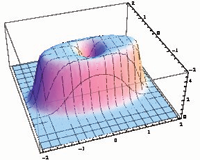We briefly review the solution of three ensembles of non-Hermitian random matrices generalizing the Wishart-Laguerre (also called chiral) ensembles. These generalizations are realized as Gaussian two-matrix models, where the complex eigenvalues of the product of the two independent rectangular matrices are sought, with the matrix elements of both matrices being either real, complex or quaternion real. We also present the more general case depending on a non-Hermiticity parameter, that allows us to interpolate between the corresponding three Hermitian Wishart ensembles with real eigenvalues and the maximally non-Hermitian case. All three symmetry classes are explicitly solved for finite matrix size NxM for all complex eigenvalue correlations functions (and real or mixed correlations for real matrix elements). These are given in terms of the corresponding kernels built from orthogonal or skew-orthogonal Laguerre polynomials in the complex plane. We then present the corresponding three Bessel kernels in the complex plane in the microscopic large-N scaling limit at the origin, both at weak and strong non-Hermiticity with M-N greater or equal to 0 fixed.

http://arxiv.org/abs/1104.5203
Mathematical Physics (math-ph); Statistical Mechanics (cond-mat.stat-mech)

## Quasi-exactly solvable quartic: real algebraic spectral locus

Alexandre Eremenko, Andrei GabrielovWe describe the real quasi-exactly solvable spectral locus of the PT-symmetric quartic using the Nevanlinna parametrization.

http://arxiv.org/abs/1104.4980
Mathematical Physics (math-ph)

## PT-Symmetric Representations of Fermionic Algebras

Carl M. Bender, S. P. Klevansky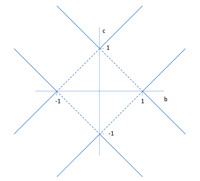A recent paper by Jones-Smith and Mathur extends PT-symmetric quantum mechanics from bosonic systems (systems for which $$T^2=1$$) to fermionic systems (systems for which $$T^2=-1$$). The current paper shows how the formalism developed by Jones-Smith and Mathur can be used to construct PT-symmetric matrix representations for operator algebras of the form $$\eta^2=0$$, $$\bar{\eta}^2=0$$, $$\eta\bar{\eta}+\bar {\eta} =\alpha 1$$, where $$\bar{eta}=\eta^{PT} =PT \eta T^{-1}P^{-1}$$. It is easy to construct matrix representations for the Grassmann algebra ($$\alpha=0$$). However, one can only construct matrix representations for the fermionic operator algebra ($$\alpha \neq 0$$) if $$\alpha= -1$$; a matrix representation does not exist for the conventional value $$\alpha=1$$.

http://arxiv.org/abs/1104.4156
Subjects: High Energy Physics – Theory (hep-th); Mathematical Physics (math-ph); Quantum Physics (quant-ph)

## Formulation of Complex Action Theory

Keiichi Nagao, Holger Bech Nielsen

We formulate the complex action theory from a fundamental level so that we can deal with a complex coordinate $$q$$ and a complex momentum $$p$$. We extend $$| q >$$ and $$| p>$$ to complex $$q$$ and $$p$$ by utilizing coherent states of harmonic oscillators. Introducing a philosophy to keep the analyticity in parameter variables of Feynman path integral, we define a modified set of complex conjugate, real and imaginary parts, hermitian conjugates and bras. They enable us to have both orthogonality and completeness relations for $$|q >$$ and $$|p >$$ with complex $$q$$ and $$p$$. We also pose a theorem on the relation between functions and operators to make it clear to some extent. Furthermore, extending our previous work \cite{Nagao:2010xu} to the complex coordinate case, we study a system defined by a diagonalizable non-hermitian bounded Hamiltonian, and show that a hermitian Hamiltonian is effectively obtained after a long time development by introducing a proper inner product. If the hermitian Hamiltonian is given in a local form, a conserved probability current density can be constructed with two kinds of wave functions.

http://arxiv.org/abs/1104.3381
Quantum Physics (quant-ph); High Energy Physics – Theory (hep-th)

## Complex correspondence principle in the modified de Broglie-Bohm quantum mechanics

Moncy V. John

The demonstration of complex correspondence principle in a recent work is noted to have the drawback that the complex paths in the classical case and complex eigenpaths in the quantum case are very much dissimilar. Also in the de Broglie-Bohm quantum mechanics, considering the harmonic oscillator coherent states, there are marked deviations from classical correspondence. In this Letter, we demonstrate this principle by showing that the complex trajectories of classical harmonic oscillator and the complex quantum trajectories in harmonic oscillator coherent state (in a modified de Broglie-Bohm approach) are identical to each other. Such congruence, which is there even for the lowest energy states, illustrates a strong correspondence principle. It does not have the defects mentioned above and is performed without resorting to any probability axiom. The example suggests that the complex classical trajectories form the limiting case of the modified de Broglie-Bohm quantum trajectories.

http://arxiv.org/abs/1104.3197
Quantum Physics (quant-ph); High Energy Physics – Theory (hep-th); Mathematical Physics (math-ph)

## Understanding complex dynamics by means of an associated Riemann surface

David Gomez-Ullate, Paolo Santini, Matteo Sommacal, Francesco Calogero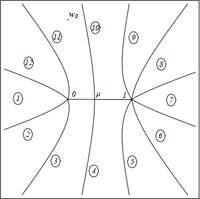We provide an example of how the complex dynamics of a recently introduced model can be understood via a detailed analysis of its associated Riemann surface. Thanks to this geometric description an explicit formula for the period of the orbits can be derived, which is shown to depend on the initial data and the continued fraction expansion of a simple ratio of the coupling constants of the problem. For rational values of this ratio and generic values of the initial data, all orbits are periodic and the system is isochronous. For irrational values of the ratio, there exist periodic and quasi-periodic orbits for different initial data. Moreover, the dependence of the period on the initial data shows a rich behavior and initial data can always be found such the period is arbitrarily high.

http://arxiv.org/abs/1104.2205
Chaotic Dynamics (nlin.CD); Dynamical Systems (math.DS); Exactly Solvable and Integrable Systems (nlin.SI)

## PT-Symmetric Sinusoidal Optical Lattices at the Symmetry-Breaking Threshold

Eva-Maria Graefe, H. F. Jones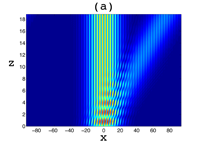The PT-symmetric potential $$V_0[\cos(2\pi x/a)+i\lambda\sin(2\pi x/a)]$$ has a completely real spectrum for $$\lambda\le 1$$, and begins to develop complex eigenvalues for $$\lambda>1$$. At the symmetry-breaking threshold $$\lambda=1$$ some of the eigenvectors become degenerate, giving rise to a Jordan-block structure for each degenerate eigenvector. In general this is expected to result in a secular growth in the amplitude of the wave. However, it has been shown in a recent paper by Longhi, by numerical simulation and by the use of perturbation theory, that for a broad initial wave packet this growth is suppressed, and instead a saturation leading to a constant maximum amplitude is observed. We revisit this problem by explicitly constructing the Bloch wave-functions and the associated Jordan functions and using the method of stationary states to find the dependence on the longitudinal distance $$z$$ for a variety of different initial wave packets. This allows us to show in detail how the saturation of the linear growth arises from the close connection between the contributions of the Jordan functions and those of the neighbouring Bloch waves.

http://arxiv.org/abs/1104.2838
Optics (physics.optics); Quantum Physics (quant-ph)

## Degrees and signatures of broken PT-symmetry in (non-uniform) lattices

Derek D. Scott, Yogesh N. Joglekar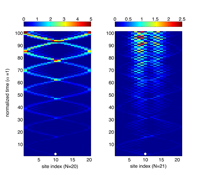We investigate the robustness of parity- and time-reversal PT-symmetric phase in an N-site lattice with position-dependent, parity-symmetric hopping function and a pair of imaginary, PT-symmetric impurities. We find that the “fragile” PT-symmetric phase in these lattices is stronger than its counterpart in a lattice with constant hopping. With an open system in mind, we explore the degrees of broken PT symmetry and their signatures in single-particle wavepacket evolution. We predict that when the PT-symmetric impurities are closest to each other, the time evolution of a wavepacket in an even-N lattice is remarkably different from that in an odd-$N$ lattice. Our results suggest that PT-symmetry breaking in such lattices is accompanied by rich, hitherto unanticipated, phenomena.

http://arxiv.org/abs/1104.1666
Quantum Physics (quant-ph); Quantum Gases (cond-mat.quant-gas)

## Nonlinearly-PT-symmetric systems: spontaneous symmetry breaking and transmission resonances

Andrey E. Miroshnichenko, Boris A. Malomed, Yuri S. Kivshar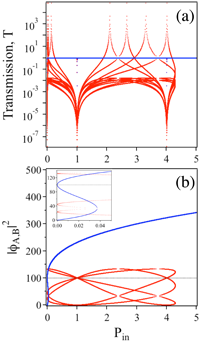We introduce a class of PT-symmetric systems which include mutually matched nonlinear loss and gain (inother words, a class of PT-invariant Hamiltonians in which both the harmonic and anharmonic parts are non-Hermitian). For a basic system in the form of a dimer, symmetric and asymmetric eigenstates, including multistable ones, are found analytically. We demonstrate that, if coupled to a linear chain, such a nonlinear PT-symmetric dimer generates new types of nonlinear resonances, with the completely suppressed or greatly amplified transmission, as well as a regime similar to the electromagnetically-induced transparency (EIT). The implementation of the systems is possible in various media admitting controllable linear and nonlinear amplification of waves.

http://arxiv.org/abs/1104.0849
Mathematical Physics (math-ph)

## On eigenvalues of the Schrödinger operator with an even complex-valued polynomial potentialIn this paper, we generalize several results of the article “Analytic continuation of eigenvalues of a quartic oscillator” of A. Eremenko and A. Gabrielov. We consider a family of eigenvalue problems for a Schr\”odinger equation with even polynomial potentials of arbitrary degree d with complex coefficients, and k<(d+2)/2 boundary conditions. We show that the spectral determinant in this case consists of two components, containing even and odd eigenvalues respectively.